Math Olympiad Test: Co-ordinate Geometry- 4

# Math Olympiad Test: Co-ordinate Geometry- 4

Test Description

## 10 Questions MCQ Test Olympiad Preparation for Class 10 | Math Olympiad Test: Co-ordinate Geometry- 4

Math Olympiad Test: Co-ordinate Geometry- 4 for Class 10 2023 is part of Olympiad Preparation for Class 10 preparation. The Math Olympiad Test: Co-ordinate Geometry- 4 questions and answers have been prepared according to the Class 10 exam syllabus.The Math Olympiad Test: Co-ordinate Geometry- 4 MCQs are made for Class 10 2023 Exam. Find important definitions, questions, notes, meanings, examples, exercises, MCQs and online tests for Math Olympiad Test: Co-ordinate Geometry- 4 below.
 1 Crore+ students have signed up on EduRev. Have you?
Math Olympiad Test: Co-ordinate Geometry- 4 - Question 1

### The coordinates of the third vertex of an equilateral triangle whose two vertices are at (3, 4), (–2, 3) are ________.

Detailed Solution for Math Olympiad Test: Co-ordinate Geometry- 4 - Question 1

Since, ΔABC is equilateral
∴ AB = AC ⇒ AB2 = AC2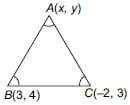⇒ (x – 3)2 + (y – 4)2
= (x + 2)2 + (y – 3)2
⇒ 5x + y – 6 = 0 ... (i)
Now, area of equilateral triangle =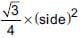∴ Area of ΔABC =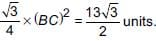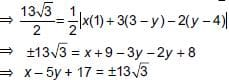⇒ x – 5y = 13√3 − 17 ...(ii)
or x – 5y = −(13√3 + 17) ...(iii)
Solving (i) and (ii), we get,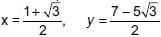Also, on solving (i) and (iii), we get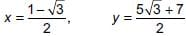Math Olympiad Test: Co-ordinate Geometry- 4 - Question 2

### The vertices of a ΔABC are A(2,1), B(6, –2), C(8, 9). If AD is angle bisector, where D meets on BC, then coordinates of D are _______.

Detailed Solution for Math Olympiad Test: Co-ordinate Geometry- 4 - Question 2

AD is the angle bisector of ∠BAC.
So, by the angle bisector theorem in ΔABC, we have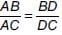...(i)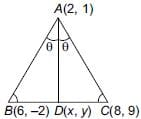Now, AB = 5 and AC = 10
∴ 1/2 = BD/DC [using (i)]
Thus, D divides BC in the ratio 1 : 2
∴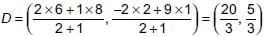Math Olympiad Test: Co-ordinate Geometry- 4 - Question 3

### Find the coordinates of the point on X-axis which are equidistant from the points (–3, 4) and (2, 5).

Detailed Solution for Math Olympiad Test: Co-ordinate Geometry- 4 - Question 3

Let P(x, 0) be the point on x-axis which is equidistant from points A(–3, 4) and B(2, 5)
Distance AP = Distance BP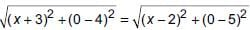⇒ (x + 3)2 + 16 = (x – 2)2 + 25
⇒ x2 + 9 + 6x + 16 = x2 + 4 – 4x + 25
⇒ 10x = 4 ⇒ x = 2/5
Co-ordinate of P (x, 0) is (2/5, 0).

Math Olympiad Test: Co-ordinate Geometry- 4 - Question 4

In what ratio is the line segment joining the points (–3, 2) and (6, 1) is divided by Y-axis?

Detailed Solution for Math Olympiad Test: Co-ordinate Geometry- 4 - Question 4

We know, co-ordinates on Y-axis is (0, y). Now, let P(0, y) divides the line segment joining the points A(–3, 2) and B(6, 1) in λ : 1.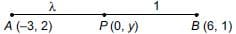Then,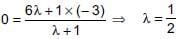∴ Required ratio = 1 : 2.

Math Olympiad Test: Co-ordinate Geometry- 4 - Question 5

Ayush starts walking from his house to office. Instead of going to the office directly, he goes to a bank first, from there to his daughter’s school and then reaches the office. If the house is situated at (2, 4), bank at (5, 8), school at (13, 14) and office at (13, 26) and coordinates are in km. What is the extra distance travelled by Ayush in reaching his office? (Assume that all distances covered are in straight lines)

Detailed Solution for Math Olympiad Test: Co-ordinate Geometry- 4 - Question 5

According to question,
Distance covered from House to Bank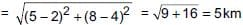Distance covered from bank to school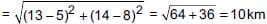Distance covered from school to office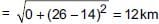∴ Total distance covered by Ayush = 5 + 10 + 12 = 27 km
Also, Distance covered directly from House to office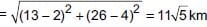∴ Required distance = 27 – 11√5 = 2.40 km

Math Olympiad Test: Co-ordinate Geometry- 4 - Question 6

If the centroid of the triangle formed by the points (a, b), (b, c) and (c, a) is at the origin, then a3 + b3 + c3 =

Detailed Solution for Math Olympiad Test: Co-ordinate Geometry- 4 - Question 6

Since, centroid of triangle formed by (a, b), (b, c) and (c, a) is at the origin,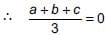⇒ a + b + c = 0 ...(i)
Also, a3 + b3 + c3 – 3abc = 1/2 [a + b + c] × [(a – b)2 + (b – c)2 + (c – a)2]
⇒ a3 + b3 + c3 – 3abc = 0 (from (i))
⇒ a3 + b3 + c3 = 3abc

Math Olympiad Test: Co-ordinate Geometry- 4 - Question 7

DIRECTION: Students of a school are standing in rows and columns in their playground for drill practice. A, B, C and D are the position of the four students as shown in the figure.Q. If Mohit wants to stand in such a way that he is equidistance from each of the four students A, B, C and D then what are the coordinate of his position?

Detailed Solution for Math Olympiad Test: Co-ordinate Geometry- 4 - Question 7

Mohit’s position will be (7, 5).

Math Olympiad Test: Co-ordinate Geometry- 4 - Question 8

DIRECTION: Students of a school are standing in rows and columns in their playground for drill practice. A, B, C and D are the position of the four students as shown in the figure.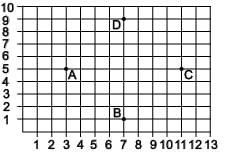Q. What is the difference of distance between AC and AD?

Detailed Solution for Math Olympiad Test: Co-ordinate Geometry- 4 - Question 8

From the given figure, Coordinates of A, B, C and D are respectively (3, 5), (7, 1), (11, 5) and (7, 9)
Distance from A to C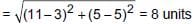Distance from A to D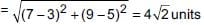∴ Required difference = 8 – 4√2 = 2.34 units

Math Olympiad Test: Co-ordinate Geometry- 4 - Question 9

To raise social awareness about t he hazards of smoking, a school decided to start “No smoking campaign”. A student is asked to prepare a campaign banner in the shape of a triangle shown in the figure. If cost of 1 cm2 of banner is ₹2, find the cost of the banner.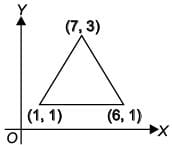Detailed Solution for Math Olympiad Test: Co-ordinate Geometry- 4 - Question 9

Area of banner
1/2= | [7(1− 1) + 1(1− 3) + 6(3 − 1)] = 5 cm2
Cost of 1 cm2 of banner = ₹2
∴ Cost of 5 cm2 of banner = 2 × 5 = ₹10

Math Olympiad Test: Co-ordinate Geometry- 4 - Question 10

A well planned locality, has two straight roads perpendicular to each other. There are 5 lanes parallel to Road - I. Each lane has 8 houses as seen in figure. Chaitanya lives in the 6th house of the 5th lane and Hamida lives in the 2nd house of the 2nd lane. What will be the shortest distance between their houses?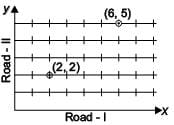Detailed Solution for Math Olympiad Test: Co-ordinate Geometry- 4 - Question 10

According to question,
Coordinates of Chaitanya’s house is (6, 5)
Coordinates of Hamida’s house is (2, 2)
∴ Shortest distance between their houses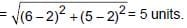## Olympiad Preparation for Class 10

11 videos|36 docs|201 tests
 Use Code STAYHOME200 and get INR 200 additional OFF Use Coupon Code